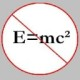You are currently browsing the tag archive for the ‘emil artin’ tag.

1. Notions of Set Theory

Artin introduces a concept that is referred to as the canonical factoring of a map (function). The basic idea is that any function$f$ can be factored into three functions$f_1, f_2$ and$f_3$ in a somewhat unique way:$f = f_3 f_2 f_1$, where$f_1$ is onto,$f_2$ is a bijection, and$f_3$ is an injection. The construction of these three functions is done in a canonical, or natural, way that doesn’t require the use of objects outside the domain and/or range of$f$.

Let$S$ be some non-empty set. If$f$ is a function from$S$ into a set$T$, then we write$f: S \to T$.

Suppose$f: S \to T$ and$g: T \to U$. Then, we can form a composite function$g \circ f: S \to U$ defined by$(g \circ f)(s) = g(f(s))$ for all$s \in S$. The associative law holds trivially for composition of functions.

Further, if$S_0 \subset S$, then the set of all the images of elements of$S_0$, denoted by$f(S_0)$, is called the image of$S_0$. In general,$f(S) \subset T$. We call the function$f$ onto whenever$f(S) = T$.

Now, let us partition the set$S$ into equivalence classes such that$s_1, s_2 \in S$ are in the same equivalence class iff$f(s_1) = f(s_2)$. This partition is called the quotient set and is denoted by$S_f$.

To illustrate, suppose$S = \{ 1, 2, 3, 4\}$ and$T = \{ a, b, c, d\}$. Also, let$f: S \to T$ such that$f(1) = a, f(2) = b, f(3) = b$ and$f(4) = c$. Then, the quotient set,$S_f = \{ \{ 1\}, \{ 2, 3\}, \{ 4\}\}$.

We construct now a function$f_1: S \to S_f$ that maps each$s \in S$ to its equivalence class. It can be verified that$f_1$ is onto. So, taking the above example, we have$f_1(1) = \{ 1 \}$,$f_1(2) = \{ 2, 3\}$,$f_1(3) = \{ 2, 3\}$ and$f_1(4) = \{4\}$.

Next, we construct a function$f_2: S_f \to f(S)$ where each element (which is an equivalence class) of$S_f$ is mapped to a$t \in T$ where each$t$ is the image of the members of the equivalence class. Recall that$s_1, s_2 \in S$ are in the same equivalence class iff$f(s_1) = f(s_2)$. Therefore,$f_2$ is one-to-one and onto. Continuing with our above example, we have$f_2(\{ 1\}) = a$,$f_2(\{ 2, 3\}) = b$ and$f_2(\{ 4\}) = c$.

And, finally, we construct a trivial function$f_3: f(S) \to T$ where$f_3(t) = t$ for each$t \in f(S)$. Note that$f_3$ is not an identity because it maps a subset,$f(S)$, into a possibly larger set,$T$, i.e.$f_3$ is an identity iff$f$ is onto. In general,$f_3$ is one-to-one and into (i.e. an injection.)

Thus, if$f(s) = t$, we note that$f_3 f_2 f_1 (s) = t$ for every$s \in S$.

And, so,$f = f_3 f_2 f_1$.

Once again,$f_1$ is onto,$f_2$ is a bijection, and$f_3$ is an injection.

It looks like it doesn’t make much sense to factor$f$ the way we did above, but we will explore more of this with respect to group homomorphisms in my next post.

Ok, I got a copy of Emil Artin‘s Geometric Algebra from the library a couple of days ago, and a careful reading of some of the parts from the first chapter has convinced me even more now that one should indeed learn mathematics from the masters themselves!

The subject employs concepts/theorems/results from set theory, vector spaces, group theory, field theory and so on, and centers around the foundations of affine geometry, the geometry of quadratic forms and the structure of the general linear group. The book also deals with symplectic and orthogonal geometry and also the structure of the symplectic and orthogonal groups.

I intend to blog on this subject for as long as I can, and this will be my first serious project for now. There are some neat things I learned in chapter I which basically deals with preliminary notions. The actual text begins from chapter II, which is titled Affine and Projective Geometry. But, chapter I has some cool techniques too, and I wish to share them in my subsequent posts.

### Blog Stats

• 349,162 hitsprof dr drd horia or… on My first postprof drd horia orasa… on My first postprof dr mircea orasa… on Inequality with lognotedscholar on Self-referential Paradoxes, In…prof dr mircea orasa… on Inequality with logprof dr mircea orasa… on Inequality with logprof dr mircea orasa… on 2010 in reviewkenji on Basic category theory, Iprof dr mircea orasa… on Solution to POW-10: Another ha…prof drd horia orasa… on Continued fraction for eprof drd horia orasa… on Inequality with logprof dr mircea orasa… on Solution to POW-12: A graph co…prof dr mircea orasa… on The Character of Physical…prof dr mircea orasa… on Milk and Tea puzzleprof drd horia orasa… on Inequality with log